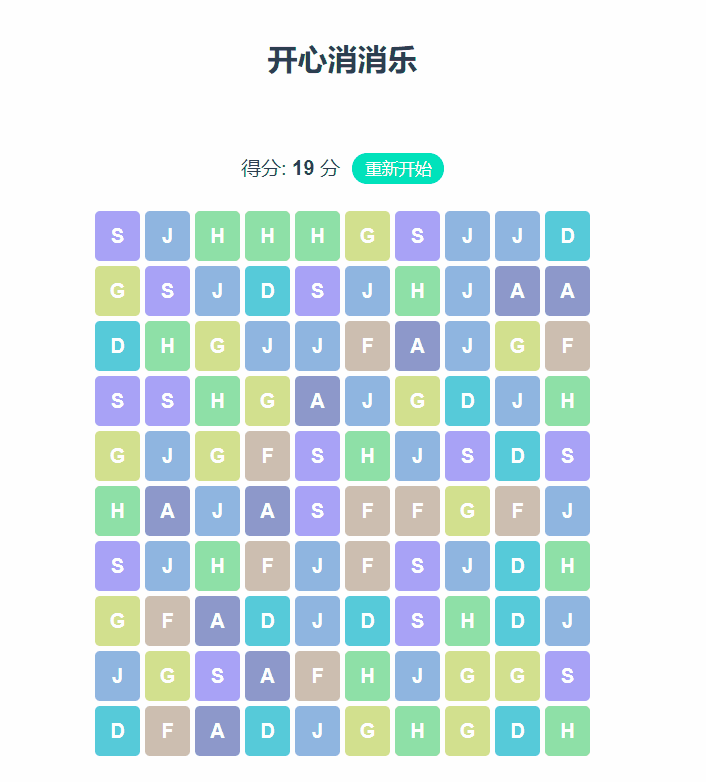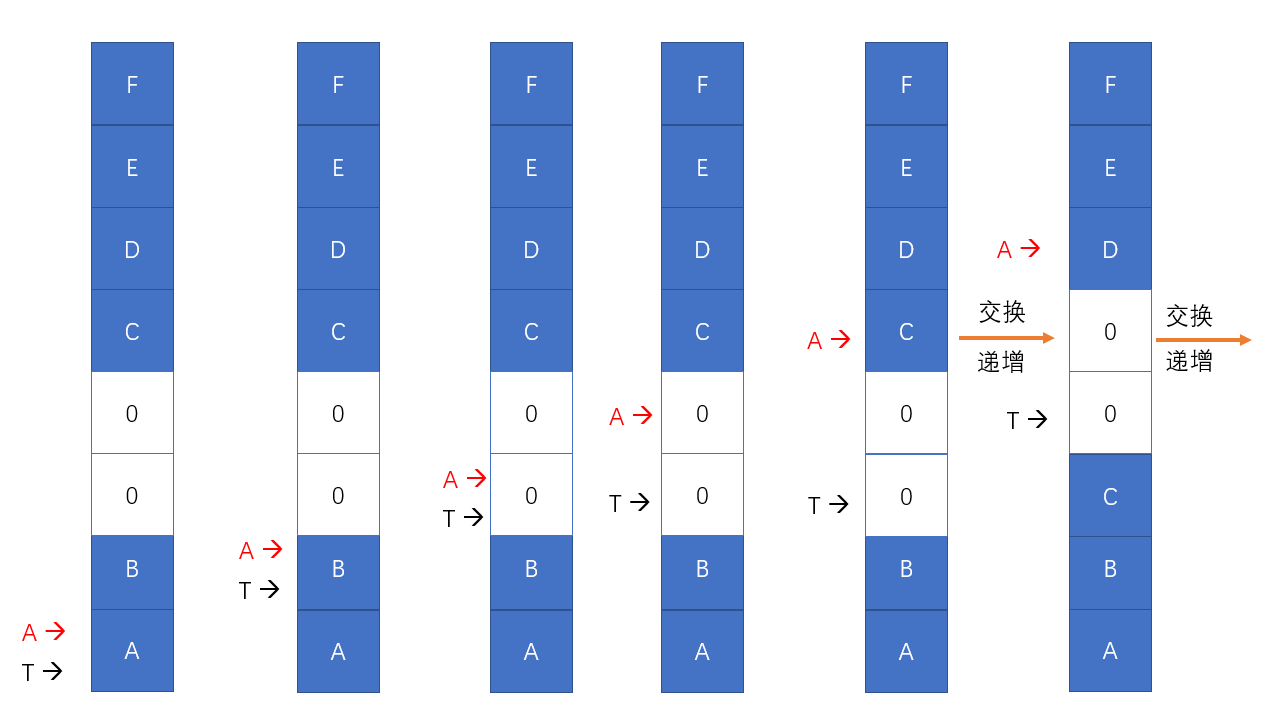# Vue实现开心消消乐游戏算法_白泊_前端开发者```<div
v-for="(item, index) in squareData"
:key="index"
class="row">
<div
v-for="(_item, _index) in item"
:key="_index"
class="square"
:class="_item"
@mousedown="dragStart(index, _index)"
@mouseup="dragEnd">
{{_item}}
</div>
</div>```

```.square.A{
background-color: #8D98CA;
}
.square.S{
background-color: #A9A2F6;
}
/*其余操作相同*/```

```@keyframes jitter {
from, 50%, to {
transform: rotate(0deg);
}
10%, 30% {
transform: rotate(10deg);
}
20% {
transform: rotate(20deg);
}
60%, 80% {
transform: rotate(-10deg);
}
70% {
transform: rotate(-20deg);
}
}
/* 只要是用户点击不动，动画就不会停止 */
.square:active{
animation-name: jitter;
animation-duration: 0.5s;
animation-iteration-count: infinite;
}``````clear(): void {
const m: number = 10;
const n: number = 10;
while (true) {
const del: any[] = [];
for (let i: number = 0; i < m; i++) {
for (let j: number = 0; j < n; j++) {
if (this.squareData[i][j] === '0') {
continue;
}
let x0: number = i;
let x1: number = i;
let y0: number = j;
let y1: number = j;
while (x0 >= 0 && x0 > i - 3 && this.squareData[x0][j] === this.squareData[i][j]) {
--x0;
}
while (x1 < m && x1 < i + 3 && this.squareData[x1][j] === this.squareData[i][j]) {
++x1;
}
while (y0 >= 0 && y0 > j - 3 && this.squareData[i][y0] === this.squareData[i][j]) {
--y0;
}
while (y1 < n && y1 < j + 3 && this.squareData[i][y1] === this.squareData[i][j]) {
++y1;
}
if (x1 - x0 > 3 || y1 - y0 > 3) {
del.push([i, j]);
}
}
}
if (del.length === 0) {
break;
}
this.score += del.length;
for (const square of del) {
this.\$set(this.squareData[square], square, '0');
}
for (let j: number = 0; j < n; ++j) {
let t: number = m - 1;
for (let i: number = m - 1; i >= 0; --i) {
if (this.squareData[i][j] !== '0') {
[this.squareData[t][j], this.squareData[i][j]] = [this.squareData[i][j], this.squareData[t][j]];
t -= 1;
}
}
}
}
},```

```if (this.score <= 0) {
if (confirm('分数用光了哦~~')) {
this.init();
} else {
this.init();
}
}```

» 本文来自：前端开发者 » 《Vue实现开心消消乐游戏算法_白泊_前端开发者》
» 本文链接地址：https://www.rokub.com/70895.html
» 您也可以订阅本站：https://www.rokub.com
64K

• 回顶